# Mini Bros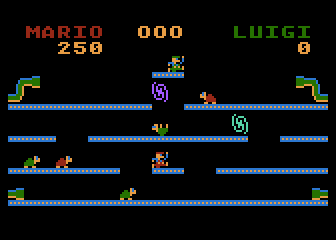This is a little game for the 2019 NOMAM's BASIC 10-liners Contest. This program fits in the EXTREM-256 category, and it was written using FastBasic 3.4 for the 8-bits ATARI XL/XE. Development started on 2018-05-09, and it took 5+5 days. The final version's date is 2019-05-28.

UPDATE: It obtained the 1st place of 18 entries in the category.

## Description

Flip the tortoises by hitting them from the bottom and kick them before they bite you. For 1 or 2 players.

## InstructionsMario and Luigi must keep clean the zone.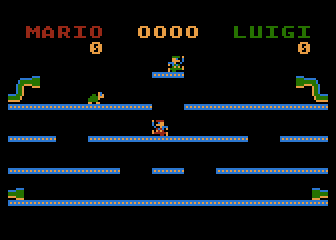Use the joystick to move to the left or to the right and avoid the walking tortoises.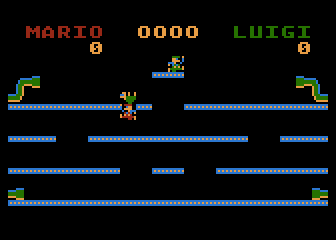Use the fire button to jump and flip tortoises by hitting them from below.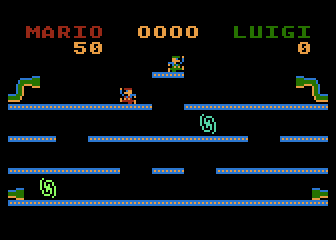Flipped tortoises can be kicked to keep the zone clean.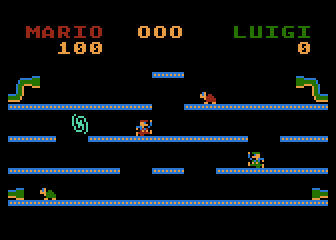If you flip again a tortoise or you did not kick a flipped one soon, it will get angry and run after you!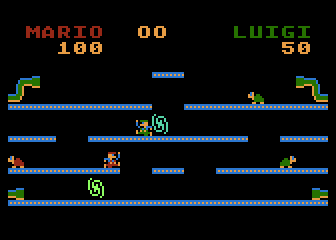Avoid the lethal fireballs. You cannot destroy them.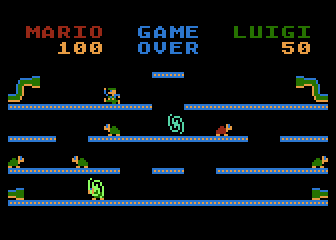Mario and Luigi can be hit and recover only 3 times in total. At the fourth hit, the game is over.

## Development of the game

I saw a new port of Mario Bros for the Sinclair ZX Spectrum called "Pietro Bros" and I thought that it could be interesting to try it as a BASIC tenliner. I drawed a prototype in a workbook for ANTIC mode 5 (4x8, double scan lines pixels, 40x12 bytes screen, or 20x12 double-bytes screen), in a very similar way I did it before in my Centipede clone called "Decipede".

I had to change a bit the playfield, but still giving the look&feel of the original game, in order to simplify the movement of the "sprites", which would be implemented using 2 bytes (8x8 pixels) and DPOKE to move them over the playfield.

As it was done with "Pietro Bros", I wanted to give credits to Mario's brother Luigi, and then called my game as "Luigi Bros", so the first version of the sprites were done in green as the main color. I used Matosimi's Atari FontMaker v1.3.5 to design the sprites.

After a couple of hours, I got a working prototype in FastBasic v3.4 with the simplified playfield and Luigi moving and jumping around it. The algorithm was so simple that I changed it to manage two players at the same time, allowing me to add Mario as a second player, just using the 4th color of the ANTIC mode 5 by enabling the bit 7 of the sprite bytes, so green turned into red when inverse video was used for the same font. The cost in bytes of code was minimal: some scalar variables for screen position and state of the player were turned into arrays and a FOR-NEXT loop was added to iterate between them.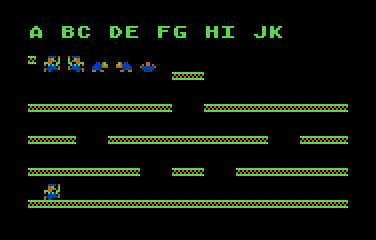Luigi moving around the playfield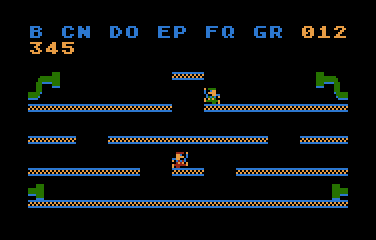Luigi and Mario

The next step was to add the tortoise's logic, and I decided to use the same arrays than the players to save space. Another FOR-NEXT loop allowed to manage many, many tortoises, one at a time, but every time I increased the number of them, the speed of the game decreased, so I had to find the right number to to let the game be playable and quite difficult at the same time.

Then, I had to add the logic for the interaction of the players and the tortoises, manage the lives and the scores, and I was running out the available space for the code. I did some optimizations and the game was working OK in only 8 lines and a half after many hours of coding/debugging/playing though 5 days. At that time, I decided to switch the players and to clone the early stage of Mario Bros with a simplification: only one endless stage with collaborative work of the two players, sharing lives but not the score.Tortoises walking down though the playfieldThe first working game

However, the game was not complete. I had one line and a half (about 400 bytes) to add at least one of the following things: sound effects, the super pill and the fireballs. It took many hours through 4 more days to add fireballs and sound within the available space.

The fireballs were implemented using P/M graphics. As there were 4 levels where Mario and Luigi could walk, I place one player to each level and moved them horizontally in a random way, but not in another FOR-NEXT loop. This time, I moved one flame at a time on every iteration of the main game loop. In the final version, each flame appears randomly on any horizontal position of its level, and moves continuously to any of its sides, wrapping around on the playfield like the players, and disappearing after some random time. Note that there is only one safe place in the playfield: the starting platform at the top.

On each iteration, I was adjusting the bitmaps of the spites and the playfield, the max number of tortoises and the color palette. I wanted to add extra bitmaps to the sprites in order to animate them, but there was no more room for that... :-(Adding fireballs to the gameFinal version

Get the MINIBROS.ATR file and set it as drive 1 in a real Atari (or emulator). Turn the computer on and the game should start after the loading completes. A joystick in port 1 or 2 is required for one player, and both joysticks are required for two players playing at the same time, competitive or collaborative.

## The code

The abbreviated BASIC code is the following: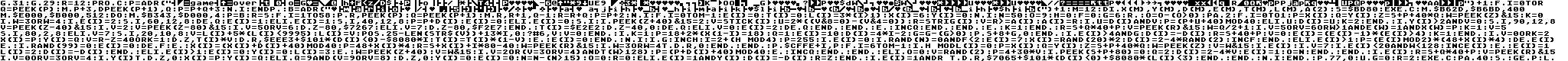The full and expanded BASIC listing is:

 ```graphics 31 ``` Wipe out memory from \$A150. Memory usage: - \$B000-B3FF: Charset - \$B400-B7FF: P/M data - \$BD6C-BD7F: Display List - \$BD80-BF60: Screen data ```graphics 29 ``` ANTIC 5 mode, 40x12, first two lines are changed to ANTIC 7 mode later ```r=12 ``` Number of configuration settings to apply (chunks). Declaration of R is required by FastBasic before its first reference in the PROC ```proc c ``` Procedure to load configuration ``` p=adr("{binary data}")+1 ``` Sequence of data blocks in the following format: - 1 byte: number of bytes in the chunk - 2 bytes: memory address to store the chunk - q bytes: chunk of data The chunks are: Game Over message, new CHBAS address (\$B000), turns the top of the DL into text (ANTIC 7), color palette (PAL), GRACTL (Players only, no missiles), GPRIOR=1, SDMCTL=2(Std)+8(player DMA)+0(Double line)+32(DMA), PMBASE=\$B400, Width of P0-P3=normal, Player 0 bitmap (fireballs), and charset for sprites from 98 to 114 (\$62-\$72). ``` for i=0 to r q=peek(p) move p+3,dpeek(p+1),q p=p+q+3 next i ``` This loop iterates over the data, moving Q bytes to the corresponding address for the chunk. ```endproc ``` ```b=adr("{binary data}")+1 ``` Compressed playfield ```m=12 ``` Max number of sprites, numbered as: 0-1=players, 2-5:fireballs, 6-11:tortoises ```dim x(m),y(m),d(m),e(m),t(m),l(m),a(2) ``` Arrays to manage each sprite: X: Horizontal coordinate of the sprite (0-38, even values) Y: Vertical coordinate of the sprite (1 to 9=top to bottom, 0=out of screen) D: Current moving direction (-2=left, 2=right) E: Status of the sprite: - player: 0=waiting 1=jumping(H) 2=jumping(V) 3+=resuming(timer) - tortoise: 0=off 1=walking 2+=flipped(timer) - fireball: 0=off 1=active 2+=appearing(timer) T: Saves what was over the head of a player when he jumped (floor or nothing) L: - player: score - tortoise: current speed 3=slow 2=fast A: Recalls if trigger for the player was already pressed, trigger works only when it is just pressed ```s=\$BD80 ``` Base of screen data ```exec c ``` Performs the configuration (all chunks) ```move \$B62D,\$B6BD,400 ``` Copies P0 to P1-P3, 16 bytes later (32 scan lines) each with respect to the previous ```move \$E000,\$B000,512 ``` Copies the first half of the charset from ROM to be used by ANTIC 7 lines ```do ``` MAIN LOOP ``` move \$B343,\$D000,4 ``` Removes P/M from screen moving them to the far right ``` p=b r=s for i=1 to 58 poke r,peek(p) q=peek(p+1) move r,r+1,q-1 r=r+q p=p+2 next i ``` Unpacks the playfield over the screen memory ``` for i=0 to m-1 e(i)=0 t(i)=0 l(i)=3*(i>1) x(i)=6 y(i)=0 next i ``` Initializes the arrays ``` n=50 ``` Delay to release tortoises ``` o=9 ``` Current release counter, release tortoises at O=0 ``` h=0 ``` Step counter, this is the timing for tortoises and fireballs ``` f=0 ``` Current number of fireballs (max 2 of 4) ``` g=6 ``` Number of remaining lives (first 2 are lost at game start just to place the players) ``` repeat ``` GAME LOOP ``` o=o-(o>0) ``` Decrease the tortoise release counter ``` pause 2 ``` Game delay... not so difficult! ``` for i=0 to 1 ``` Players management ``` p=x(i) q=y(i) z=s+p+40*q ``` Current position of the player in the playfield ``` w=peek(z)&15 k=0 ``` Checks if it has not been replaced by a tortoise ``` if w=3 or w=4 ``` Player still on screen? ``` if e(i)=2 sound i,60,12,8 dec q e(i)=1 ``` Player was jumping (phase 1: vertical) ``` elif e(i)=1 sound i,40,12,8 p=p+d(i) e(i)=0 ``` Player was jumping (phase 2: horizontal) ``` elif e(i)=0 sound i ``` ``` if peek(z+40)&15=2 ``` Has the player his feet on the floor? ``` v=stick(i) u=2*((v&8=0)-(v&4=0)) ``` He could walk, check joystick for horizontal move ``` r=strig(i) v=r>=a(i) a(i)=r ``` He could jump, check if the trigger was just pressed ``` if u=d(i) and v ``` Not jumping nor turning direction ``` p=(p+u+40) mod 40 ``` Move horizontally to the same direction (with screen warp) ``` elif u d(i)=u k=2 endif ``` Change walking direction ``` if y(i)>2 and v=0 sound i,90,12,8 ``` Jumps ``` v=peek(z-40)&15 ``` What is over the player? ``` if v=2 ``` Platform ``` v=peek(z-80)&15 ``` What is over the platform? ``` if v=5 or v=6 dpoke z-80,\$7267 ``` Tortoise, flip it! ``` elif v=7 dpoke z-80,\$F2E7 endif ``` Tortoise on its back, signal to make it walking again, but faster ``` t(i)=\$E2E2 dpoke z-40,0 dec q ``` Remember that a piece of platform will be replaced by the player ``` elif v=0 e(i)=2 dec q endif endif ``` Nothing, continue the jumping sequence ``` else inc q endif endif ``` There is no platform under hes feet, try to fall ``` else k=1 endif ``` Died? ``` r=s+q*40+p ``` Compute next position of the player ``` v=peek(r)&15 w=q/2+1 ``` What is in that position? ``` if v=5 or v=6 or w>1 and e(w)=1 and x(w)=p k=1 v=5 sound i,80,2,8 ``` Killed if there is a tortoise or a fireball ``` elif v=7 sound i,20,10,8 ``` Kick a flipped tortoise ``` v=l(i)+5*(l(i)<9995) l(i)=v position 25-len(str\$(v))+13*i,0 ? #6,v v=0 endif ``` Compute the score, max score is 99950 ``` if k=1 p=18+2*(x(1-i)=18) q=1 e(i)=10 d(i)=4*i-2 ``` If the player was killed, sets the new coordinates at the start position ``` g=g-(g>0) ``` Decrease lives ``` poke s+8+g,0 endif ``` Remove one life from the screen ``` if e(i)>4 and g d(i)=-d(i) r=s+40+p v=0 e(i)=(e(i)-1)*(e(i)>4) k=1 endif ``` Player is appearing in one of the starting positions ``` if v=0 or k=2 x(i)=p y(i)=q v=r-z=40 or k=1 dpoke z,t(i)*v dpoke r,\$EEE3+\$101*(d(i)<0)-\$8080*i t(i)=t(i)*(1-v) else e(i)=0 endif next i ``` Move the player to the new location, restoring the old one if it was a floor over his head ``` if g ``` Are there remaining lives? ``` inc h ``` Increase the step counter ``` i=2+(h mod 4) ``` Selects current fireball ``` p=255 ``` Sets default position out of screen ``` if e(i)=0 ``` Is flame off? ``` if rand(n)=0 and f<2 e(i)=7 x(i)=rand(20)*2 d(i)=2-4*rand(2) inc f endif ``` Turns on the flame if it is possible ``` elif e(i)>1 p=(e(i) mod 2)*(48+x(i)*4) dec e(i) ``` Blinking fireball while turning it on ``` else ``` The fireball was already on... ``` if rand(99)=0 e(i)=0 dec f ``` Turn it off? ``` else x(i)=(x(i)+d(i)+40) mod 40 p=48+x(i)*4 ``` Move it one step ``` r=s+x(i)+i*80-40 w=peek(r)&15 ``` Check if there is a player in the new location ``` if w=3 or w=4 then dpoke r,0 endif endif ``` A player was detected... kill him! ``` poke \$CFFE+i,p ``` Sets the new location of the current fireball ``` for i=6 to m-1 ``` Management of the tortoises ``` if h mod l(i)=0 ``` Should the current tortoise move? ``` p=x(i) q=y(i) z=s+p+40*q w=peek(z) v=w&15 ``` Current position of the tortoise in the playfield ``` if e(i) ``` If current tortoise is alive ``` if v=7 ``` Is the tortoise on its back? ``` if e(i)<20 and w<128 inc e(i) ``` Still waiting... ``` else e(i)=1 l(i)=2 d(i)=-d(i) endif ``` The tortoise starts to run ``` elif e(i)>1 e(i)=0 y(i)=0 l(i)=3 ``` Not there... it was kicked! ``` else w=peek(z+40) v=w&15 ``` Calculate next location ``` if v=2 or (v=3 or v=4) and t(w>128) ``` Over the platform (or the player jumping to it)? ``` p=(p+d(i)+40) mod 40 ``` Move horizontally ``` else inc q endif endif ``` Falling down ``` elif o=0 ``` Releases a tortoise ``` v=rand(2) p=4+30*v ``` Selects a pipe ``` if peek(s+p+80)=0 q=2 d(i)=2-4*v e(i)=1 o=n endif endif ``` Release a tortoise it only if the pipe is free ``` if e(i) r=s+q*40+p v=peek(r)&15 ``` If the tortoise is alive ``` if v=0 or v=3 or v=4 ``` If the new position is free or there is a player ``` if y(i) then dpoke z,0 x(i)=p y(i)=q ``` Clean the previous location ``` elif q=9 and (v=9 or v=8) ``` Has reached an exit pipe? ``` dpoke z,0 y(i)=0 e(i)=0 n=n-(n>15) o=0 r=0 ``` Prepare to release it from the top pipes ``` elif e(i)=1 and y(i) ``` Did it hit an obstacle? ``` d(i)=-d(i) r=z endif ``` Keeps same location, but change walking direction ``` if e(i)=1 and r then dpoke r,\$7065+\$101*(d(i)<0)+\$8080*(l(i)<3) ``` Put a living tortoise in the new location ``` endif endif ``` ``` next i ``` ``` endif ``` ``` poke 77,0 ``` Disables ATRACT mode ``` until g=0 ``` End of game loop ``` r=2 exec c ``` Print the GAME OVER message (using first two configs) ``` pause 40 sound ``` Waits and stops the killed sound ``` get p ``` Press any key to start a new game ```loop ``` End of main loop